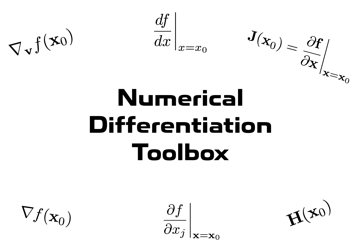# Numerical Differentiation Toolbox Documentation## Opening this documentation using the MATLAB Command Window.

```doc_NDT
```

in the Command Window. To open the documentation of a specific function with name function_name from the Command Window, type

```doc_NDT function_name
```

To open the PDF file with the technical documentation (Numerical_Differentiation.pdf) from the Command Window, type

```doc_NDT tech
```

## Installation

• docs → Contains the HTML documentation needed by GitHub to deploy the online version of the toolbox documentation.
• INSTALL → Contains the toolbox installer (Numerical Differentiation Toolbox.mltbx).
• README.md → Markdown documentation for GitHub repository.
• Technical Documentation → Contains the technical documentation (Numerical_Differentiation.pdf) for the various algorithms.
• tests → Code for unit tests (requires the Simple Unit Testing Toolbox).
• toolbox → Contains all the functions specific to this toolbox.
• toolbox/doc → Contains a copy of all the files and functions needed to open both the toolbox documentation and the technical documentation directly from the MATLAB command window using the doc_NDT function.

To install as a toolbox, simply open "Numerical Differentiation Toolbox.mltbx" in the "INSTALL" folder. MATLAB will automatically perform the installation and add all the functions included in the toolbox to the MATLAB search path.

Alternatively, all of the functions in the "toolbox" folder can be used independently.

## Complex-Step Differentiation Functions

• iderivative Derivative of a univariate, vector-valued function using the complex-step approximation.
• ipartial Partial derivative of a multivariate, vector-valued function using the complex-step approximation.
• igradient Gradient of a multivariate, scalar-valued function using the complex-step approximation.
• idirectional Directional derivative of a multivariate, scalar-valued function using the complex-step approximation.
• ijacobian Jacobian of a multivariate, vector-valued function using the complex-step approximation.
• ihessian Hessian of a multivariate, scalar-valued function using the complex-step approximation.

## Complexified Functions

• iabs Absolute value ("complexified" version of the abs function).
• iatan2 Four-quadrant inverse tangent in radians ("complexified" version of the atan2 function).
• iatan2d Four-quadrant inverse tangent in degrees ("complexified" version of the atan2d function).
• idot Vector dot product ("complexified" version of the dot function).
• imax Maximum of two numbers ("complexified" version of the max function).
• imin Minimum of two numbers ("complexified" version of the min function).
• imod Remainder after division ("complexified" version of the mod function).
• inorm 2-norm of a vector ("complexified" version of the norm function).

## Central Difference Differentiation Functions

• cderivative Derivative of a univariate, vector-valued function using the central difference approximation.
• cpartial Partial derivative of a multivariate, vector-valued function using the central difference approximation.
• cgradient Gradient of a multivariate, scalar-valued function using the central difference approximation.
• cdirectional Directional derivative of a multivariate, scalar-valued function using the central difference approximation.
• cjacobian Jacobian of a multivariate, vector-valued function using the central difference approximation.
• chessian Hessian of a multivariate, scalar-valued function using the central difference approximation.

## Forward Difference Differentiation Functions

• fderivative Derivative of a univariate, vector-valued function using the forward difference approximation.
• fpartial Partial derivative of a multivariate, vector-valued function using the forward difference approximation.
• fgradient Gradient of a multivariate, scalar-valued function using the forward difference approximation.
• fdirectional Directional derivative of a multivariate, scalar-valued function using the forward difference approximation.
• fjacobian Jacobian of a multivariate, vector-valued function using the forward difference approximation.
• fhessian Hessian of a multivariate, scalar-valued function using the forward difference approximation.

## Warnings/Disclaimers

• Whenever a transpose is used in a function that is being differentiated using the complex-step approximation, special care must be taken to use the non-conjugate transpose (.') instead of the conjugate transpose (') → see Section 3.3.1 of the technical documentation.
• There are some special cases of functions where the complex-step approximation will not work directly; for example, trying to differentiate functions using MATLAB's atan2 or abs would result in errors. We can "complexify" these functions to make them suitable for use with the complex-step approximation; in this toolbox, we have included iabs, iatan2, iatan2d, idot, imax, imin, imod, and inorm in src/complexified. However, more complexified functions can be found (programmed in Fortran) at https://mdolab.engin.umich.edu/misc/files/complexify.f90.
• Most differentiable functions can be used with the various functions of this toolbox. Functions that result in errors when using the complex-step approximation functions are summarized in Section 3.4.2 of the technical documentation.
• The functions in this toolbox can only perform first-order derivatives.
• All of the complex-step approximation functions are (generally) accurate to double precision with the exception of ihessian. An alternative that may perform better is the hessian function provided by the Adaptive Robust Numerical Differentiation toolbox.
• No error estimates are provided.